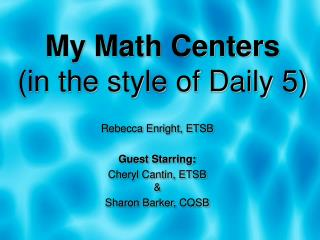DownloadDownload PresentationMy Math Centers (in the style of Daily 5)

# My Math Centers (in the style of Daily 5)

Télécharger la présentation## My Math Centers (in the style of Daily 5)

- - - - - - - - - - - - - - - - - - - - - - - - - - - E N D - - - - - - - - - - - - - - - - - - - - - - - - - - -
##### Presentation Transcript

1. My Math Centers (in the style of Daily 5) Rebecca Enright, ETSB Guest Starring: Cheryl Cantin, ETSB& Sharon Barker, CQSB

2. Quick Poll • Have you heard of D5 before? • Do you do D5 ELA in your class? • What cycle do you teach?

3. Advantages of D5 Centers • Great for a split cycle • Allows for differentiation • Small group instruction • Quick, on the spot assessment FOR learning • Active engagement • Familiar structure • Independent students

4. Transitions: Music Box

5. Timing Option 1:Two 30 minute periods

6. Timing Option 2: Three 20 minute periods

7. Storage

8. Introducing: Game Days! • Learn new games • Learn where to put games away • Practice quiet voices • Practice transitions • Practice group work • Build stamina • Build independence

9. Time to Practice!

10. Vocabulary:

11. Frayer Model

12. Arithmetic: Addition At least At most Decreasing order Difference Digit Dividend Division Divisor Equal to Equality Equation Even number Expanded form Factor Greater than Grouping Growing pattern Hundreds place Increasing order Inequality Inverse operation Less Less than Minus Missing term More Multiple Multiplication Natural number Number Number line Odd number Pattern Pattern rule Plus Product Quotient Remainder Series Sharing Subtraction Sum Tens place Term Unit Stats: Bar graph Broken line graph Pictograph Survey Table Decimals: DecimalHundredth Tenth Geometry: Cartesian plane Circle Closed straight line Convex polygon Curved line Edge Frieze patterns Line of reflection Net of a solid Non-convex polygon Ordered pair Parallel Parallelogram PerpendicularPlane Plane figure Polygon Quadrilateral Rectangle Reference system Reflection Rhombus Right angle triangle Segment Side Square Straight line Symmetric figure Tessellation Trapezoid Vertex Fractions: Denominator Equivalent fraction Equivalent part Numerators One half One quarter One third Whole Probability: Certain Chance Equally likely Impossible Less likely Likely More likely Outcome Possible Probability Random experiment Unlikely Measurement: Acute angle Area Centimeter Decimeter Depth Height Hour Length Meter Millimeter MinuteObtuse angleDay Perimeter Second Surface Unit of measure Volume Angle Right angle Width Cycle II

13. Manipulatives

14. Problem Solving

15. Ketchup & Free Choice • Ketchup- finish • Free choice • Must have finished • 3 Puzzles and Problems • 3 Vocabulary terms

16. Website:

17. Thank youFor more information: Rebecca Enright enrightr@ped.etsb.qc.camsenrightsclass.pbworks.com Cheryl Cantin cantinc@etsb.qc.ca